DEFEND FOR THE POOR CARBON

2.0 Antarctic Ice Core Data
5.0 Heat Balance Mechanism 6.0 Further Interpretation of Ice Core Data 7.0 Coming Interglacial Peak Period

7.0       COMING INTERGLACIAL PEAK PERIOD

From figure 6.1, it shows that the interglacial period for each cycle increases. From sub-chapter 5.0 on “Heat Balance Mechanism”, we understand that the Earth is a natural nuclear fission reactor, where the major heat-producing isotopes undergo fission process. Base on the available data from the ice cores, we can anticipate for the next peak interglacial warm period.

For each interglacial period, it is first assumed that the amount of accumulated heat required to trigger each cycle is Q. The disintegration energy of Q given as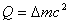... eq. 7.1

where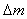= total reduction of mass of isotopes

c = the speed of light

The fission process is given by the equation of,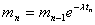... eq. 7.2

where mn = amount of mass at time n

mn-1 = amount of mass at time n-1

l = disintegration constant

tn = disintegration period from time n-1 to n

The reduction of mass at time n is given as... eq. 7.3

The amount of energy for each interglacial period is the same, hence, the mass reduction for the previous interglacial is similar to the next interglacial, we can write that,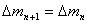... eq. 7.4

Substituting eq. 7.2 and 7.3 into eq. 7.4, we obtain,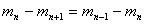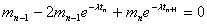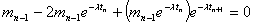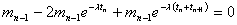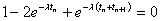... eq. 7.5

Knowing the value of tn and tn+1, via trial-and-error method, we can solve for the disintegration constant, l.

After knowing the disintegration constant, we can find out the next disintegration period, tn+1 and the time n+1 by rearrange eq. 7.5 as follow,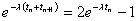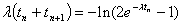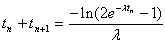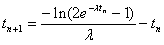... eq. 7.6Figure 7.1 – Data extracted from Vostok Ice Core. Number (1) to (3) are the three peak of interglacial warm period. These three data will be used to anticipate the next coming interglacial peak.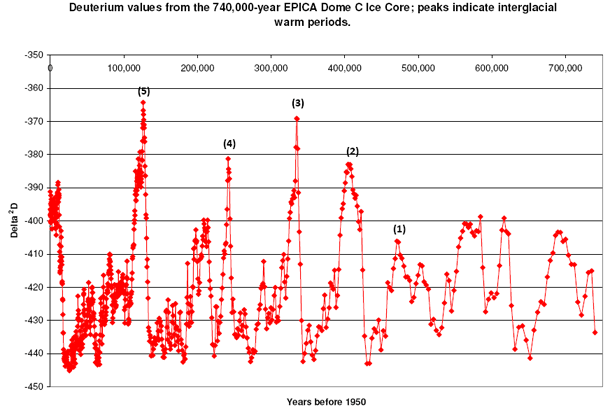Figure 7.2 – Data extracted from EPICA Dome C Ice Core. number (1) to (5) are the five peaks of interglacial warm period. These five data will be used to anticipate the next coming interglacial peak.

Figure 7.1 and 7.2 shows the Vostok and the EPICA Dome C Ice Core data. Using eq. 7.5 and 7.6, we calculate and tabulate the values in table 7.1 and 7.2 for the Vostok and EPICA Dome C Ice Core respectively as follows,

 n Year BP Disintegration period, tn Disintegration constant, l Calculated tn+1 Calculated next peak year 1 323,485 - - - - 2 238,199 85,286 - - - 3 128,652 109,547 2.5833 x 10-6 153,351 -24,699

Table 7.1 – Base on the data given by Vostok Ice Core for the 3 numbers of peaks, the calculated value for the next peak is around 25,000 years from now.

 n Year BP Disintegration period, tn Disintegration constant, l Calculated tn+1 Calculated next peak year Compare to actual year 1 473,052 - - 2 408,392 64,660 - 3 334,577 73,815 1.9219 x 10-6 86,046 248,531 6994 4 241,537 93,040 2.7868 x 10-6 125,943 115,594 -10,554 5 126,148 115,389 2.0737 x 10-6 151,985 -25,837 ±10,000

Table 7.2 – Base on the data given by EPICA Dome C Ice Core for the 5 numbers of peaks, the calculated value for the next peak is around 26,000 years from now. The calculated values (shown in blue colour) for tn+1 and the peak year are closed to the recorded data (shown in red colour).

From table 7.1 and 7.2 and both Vostok and EPICA Dome C Ice Core data, the calculated disintegration constant varies from 1.9x10-6 to 2.8x10-6, this may due to the accuracy of the collected data, where the recorded peak data for both ice cores are different and also may due to the effect of solar energy. The calculated time for the next interglacial peak period is around 25,000 years from now. It is projected that the next interglacial warm peak will reach on the 25,000 years from now.

| Main page |

This website is originated on 8-April-2010,

updated on 27-April-2010.